A number of systems for wavelength dispersion measurement are produced by various manufacturers and are commerically available. The measurement methods are phase method, differential phase shift method, pulse method, interferometry method, and so on. In measuring the dispersion with these methods, it it nice to derive the wavelength difference by a differentiation after approximating the group delay with polynomial equation, called as the Sellmeier equation. Depending on a sort of optical fiber, an approximation formula used, and the number of measured wavelengths minimally required for the approximation are varied.

In the following, for reference, an approximation formula, and equations for deriving a dispersion parameter, D(λ)=dτ(λ)/dλ, a zero dispersion wavelength, λD, and a dispersion slope, sD, for some Corning optical fibers are summarized. τ(λ) is a decay time for 1 km propagation and has a unit of [ps/km]. Therefore, τ(λ) indicates an inverse of group velocity dispersion, vg, and is equivalent to β1.

(1) Normal singlemode fiber (e.g.Corning SMF-28, SMF-28e)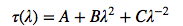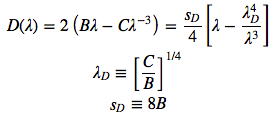(2) Dispersion shifted singlemode fiber (e.g.Corning SMF/DS, SMF-LS)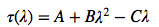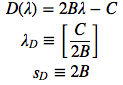(3) Other types of dispersion shifted singlemode fiber (e.g.Corning LEAF, MetroCor)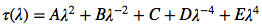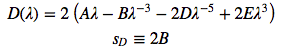In a dispersion measurement, the pulse intensity in optical fiber needs to be low in order to avoid nonlinear optical effects. The phase method, the differential phase shift method, the pulse method, and the interferometry method are briefly explain in the following texts. Each measurement method has a good or bad. It is better to select proper one out of the methods depending on a measuring objective.

### Phase method and differential phase shift method

The phase method utilizes a number of light source emitting different wavelengths. Then, light with different wavelengths is input into a measured optical fiber, and differences in arrival time of signal light among each wavelength determine a value of wavelength dispersion. Concretely, when light with a certain wavelength is modulated by a constant frequency, the phase difference between input and output is read and recorded. By repeating this procedure with the number of wavelengths required for approximation, the Sellmeier equation is completed. Additionally, by measuring delay time, β1, depending on a wavelength and differentiating β1 with respect to ω, a value of β2 is obtained.

A similar measurement method is the differential phase shift method, in which a value of wavelength dispersion is directly measured provided that D(λ) is linear. As a light source, a group of light source with a small wavelength difference is employed. The Sellmeier equation is not used for the differential phase shift method.

### Pulse method

In the pulse method, a group delay time for each wavelength in the time domain is directly measured. The approximation equation for wavelength dispersion is completed by a difference in arrival time among optical pulses with different wavelengths. A general configuration of pulse method is shown in Fig.2.18. The pulse method requires a knowledge on the delay in the testing device, and also requires an accurate calibration of device for a precise measurement. Since the pulse methods is utilizable only when pulses with different wavelengths simply propagate in a measured fiber, it has a merit of plain measurement. However, in measuring the delay time directly, a long optical fiber is necessary since a large delay time is required.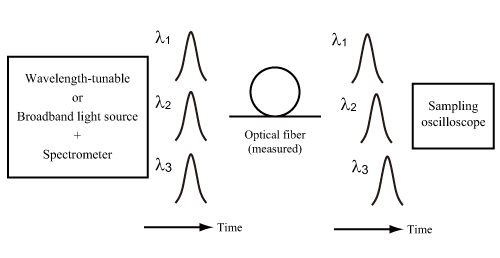Figure 2.18: Configuration of measurement system for optical fiber dispersion (pulse method)

### Interferometry method

Figure 2.19: Configuration of measurement system for optical fiber dispersion (interferometry method)

The interferometry method utilizes a coherent light source (a wideband light source such as a supercontinuum light source). A Mach-Zehnder interferometer, which is composed of reference light path and a measurement light path including a measured fiber, is used for measuring an interferometric pattern of output, and for measuring a delay time at each wavelength. Fig.2.19 schematically shows a typical configuration of interferometry method.

1.) After splitting a broadband light into a couple of beams by a beam splitter, the one propagates through a measured optical fiber, the other propagates in a reference optical path.
2.) In a reference light path, an optical delay line is arranged since the interference of beams requires a matching of propagation time difference. Generally, an optical fiber with a well-known dispersion property is employed as an optical delay line, or a spatial delay plays a role of an optical delay line. A broadband light is affected by a wavelength dispersion in propagating through a measured optical fiber, and is delayed at an interference region depending on a wavelength. Therefore, the delayed broadband light temporally overlap with light having propagated through the reference path. At a specific wavelength, the phases are matched.
3.) By measuring interference spectra with an optical spectrum analyzer with changing a length of reference optical path by a corner reflector, then measuring changes in the peak wavelength of interference, a relative delay difference at each wavelength is obtained,
4.) In case an optical fiber is used as the optical delay line, obtained data contains a difference in a propagation delay for both the measuring path and the reference path. Therefore, the data needs to be calibrated after measurements.

The interference method utilizes, as it is named, an interference, and can precisely measure a relatively small change in the dispersion. The interference method is appropriate for uses in measuring a short-length fiber. However, in case an optical fiber is used as the optical delay line, an optical fiber with a dispersion equivalent to, or larger than, a dispersion of measured fiber is not available. The interference method requires a relatively precise operation in time compared with the pulse method.